# sklearn.datasets.make_regression¶

sklearn.datasets.make_regression(n_samples=100, n_features=100, *, n_informative=10, n_targets=1, bias=0.0, effective_rank=None, tail_strength=0.5, noise=0.0, shuffle=True, coef=False, random_state=None)[source]

Generate a random regression problem.

The input set can either be well conditioned (by default) or have a low rank-fat tail singular profile. See make_low_rank_matrix for more details.

The output is generated by applying a (potentially biased) random linear regression model with n_informative nonzero regressors to the previously generated input and some gaussian centered noise with some adjustable scale.

Read more in the User Guide.

Parameters:
n_samplesint, default=100

The number of samples.

n_featuresint, default=100

The number of features.

n_informativeint, default=10

The number of informative features, i.e., the number of features used to build the linear model used to generate the output.

n_targetsint, default=1

The number of regression targets, i.e., the dimension of the y output vector associated with a sample. By default, the output is a scalar.

biasfloat, default=0.0

The bias term in the underlying linear model.

effective_rankint, default=None
If not None:

The approximate number of singular vectors required to explain most of the input data by linear combinations. Using this kind of singular spectrum in the input allows the generator to reproduce the correlations often observed in practice.

If None:

The input set is well conditioned, centered and gaussian with unit variance.

tail_strengthfloat, default=0.5

The relative importance of the fat noisy tail of the singular values profile if effective_rank is not None. When a float, it should be between 0 and 1.

noisefloat, default=0.0

The standard deviation of the gaussian noise applied to the output.

shufflebool, default=True

Shuffle the samples and the features.

coefbool, default=False

If True, the coefficients of the underlying linear model are returned.

random_stateint, RandomState instance or None, default=None

Determines random number generation for dataset creation. Pass an int for reproducible output across multiple function calls. See Glossary.

Returns:
Xndarray of shape (n_samples, n_features)

The input samples.

yndarray of shape (n_samples,) or (n_samples, n_targets)

The output values.

coefndarray of shape (n_features,) or (n_features, n_targets)

The coefficient of the underlying linear model. It is returned only if coef is True.

## Examples using sklearn.datasets.make_regression¶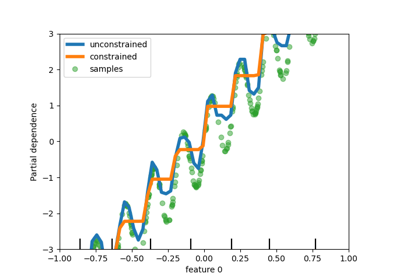Release Highlights for scikit-learn 0.23

Release Highlights for scikit-learn 0.23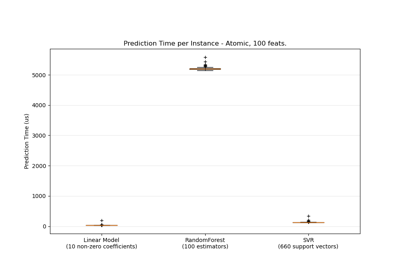Prediction Latency

Prediction Latency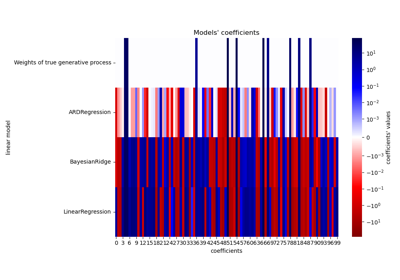Comparing Linear Bayesian Regressors

Comparing Linear Bayesian RegressorsFitting an Elastic Net with a precomputed Gram Matrix and Weighted Samples

Fitting an Elastic Net with a precomputed Gram Matrix and Weighted Samples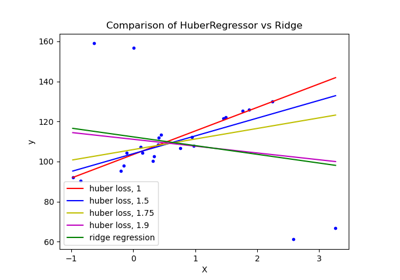HuberRegressor vs Ridge on dataset with strong outliers

HuberRegressor vs Ridge on dataset with strong outliersLasso on dense and sparse data

Lasso on dense and sparse data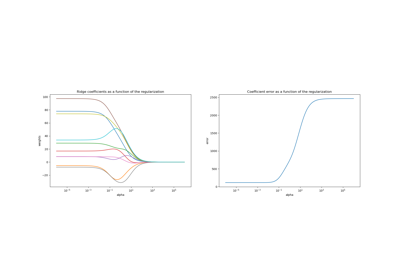Plot Ridge coefficients as a function of the L2 regularization

Plot Ridge coefficients as a function of the L2 regularization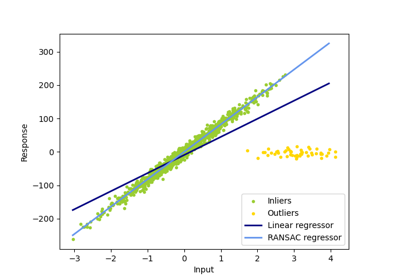Robust linear model estimation using RANSAC

Robust linear model estimation using RANSAC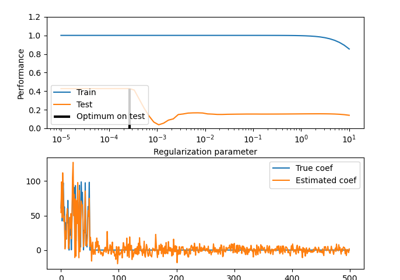Train error vs Test error

Train error vs Test error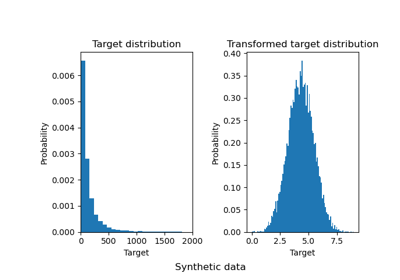Effect of transforming the targets in regression model

Effect of transforming the targets in regression model